# Using Kotlin in Android Studio 3.0 (Part 5)

### In Part 5 of this series, we continue learning about object-oriented programming, or OOP, for mobile development with Kotlin.

· Mobile Zone · Tutorial
Save
4.94K Views

## Introduction

I introduced OOP aspects for Kotlin in Part 3 and Part 4. In this article of my series, I will continue to introduce some other interesting aspects of OOP such as generic, delegation, collections, and overloading operators.

### Generic

Generics are basically used to define which types a class can hold and which type an object currently holds. An example:

``````class genericsExample < T > (input: T) {
init {
val value: T = input
}
}``````

This class now can be instantiated using any type:

``val ge1 = genericsExample<String>("Hello") val ge2 = genericsExample<Int>(4) val ge3 = genericsExample<Int?>(null)``

If we want to restrict the  `genericsExample ` to non-nullable types, we just need to do:

``````class genericsExample < T: Any > (input: T) {
init {
val value: T = input
}
}``````

If we compile previous code, we will see that  `ge3 ` throws an error: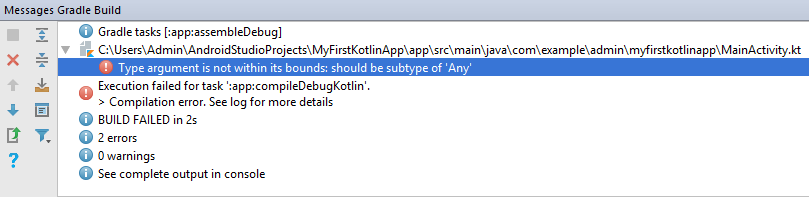Now, we try to compile the following code:

``val ge1 = genericsExample<Int>(4) val ge2 : genericsExample<Number> = ge1``

`gel2 ` will throw an error because  `Number ` is the supertype of  `Int ` and we can’t assign the generic type to any of its supertype. This problem is called covariance in Java.

And now, we try something as follows:

``val ge1 = genericsExample<Number>(4) val ge2 : genericsExample<Int> = ge1``

`gel2 ` still throws an error because we can’t assign the generic type to any of its subtype. This problem is called contravariance in Java.

Kotlin uses  `out ` for covariance and  `in ` for contravariance to make Kotlin codes more readable and easy for the developer. Using  `out ` can look like this:

``````class genericsExample < out T > (input: T) {
init {
val value: T = input
}
}``````

And the following code is OK:

``val ge1 = genericsExample<Int>(4) val ge2 : genericsExample<Number> = ge1``

Using  `in ` :

``````class genericsExample < in T > (input: T) {
init {
val value: T = input
}
}``````

The following code is OK:

``val ge1 = genericsExample<Number>(4) val ge2 : genericsExample<Int> = ge1``

We can find some practical functions such as let, with, or apply in a Kotlin standard library.

### Delegation

The delegation pattern is a really useful pattern that can be used to extract responsibilities from a class. The delegation pattern is supported natively by Kotlin, so it prevents the need for calling the delegate. Kotlin supports delegation by introducing a new keyword: " `by` ". Using the " `by` " keyword, Kotlin allows the derived class to access all the public members of an interface through a specific object. Let’s consider the following example of an interface:

``interface iShape { //properties var fillColor:String var outlineColor:String //methods fun Draw():String }``

We can use the " `by` " keyword to delegate all the public members of the  `iShape ` interface on the `s` object:

``class Shape (s:iShape):iShape by s``

We define two classes to implement the members of the  `iShape ` interface as follows:

``````class Circle: iShape {
override
var fillColor: String = ""
override
var outlineColor: String = ""
override fun Draw(): String {
return "I am a Circle. My Fill is \$fillColor and My Outline is \$outlineColor"
}
}
class Rectangle: iShape {
override
var fillColor: String = ""
override
var outlineColor: String = ""
override fun Draw(): String {
return "I am a Rectangle. My Fill is \$fillColor and My Outline is \$outlineColor"
}
}``````

Now we can either create a rectangle:

``````val rect = Shape(Rectangle()) rect.fillColor = "RED"
rect.outlineColor = "BLUE"``````

or a circle:

``````val circle = Shape(Circle()) circle.fillColor = "YELLOW"
circle.outlineColor = "GRAY"``````

Because a square is a special rectangle, we can define the Square class as follows:

``class Square:iShape by Rectangle()``

Kotlin provides some standard methods for delegation of properties in Kotlin library such as Lazy() or Observable().

Kotlin has a fixed number of symbolic operators we can easily use in any class. The way is to create a function with a reserved name that will be mapped to the symbol. Overloading these operators will increment code readability and simplicity. Examples:

``+a a.unaryPlus() a+b a.plus(b) a[i] a.get(i)``

### Collections

Unlike many languages, Kotlin distinguishes between mutable and immutable collections (lists, sets, maps, etc). Precise control over exactly when collections can be edited is useful for eliminating bugs, and for designing good APIs. An example of using the  `ArrayList ` collection:

``````val lst = ArrayList < Shape > () val rect = Shape(Rectangle()) rect.fillColor = "RED"
rect.outlineColor = "BLUE"
lst.add(rect) val circle = Shape(Circle()) circle.fillColor = "YELLOW"
circle.outlineColor = "GRAY"

### An Android Application

In this application, I have used two activities and a helper class. Two activities can contact with each other through the helper class as the following figure: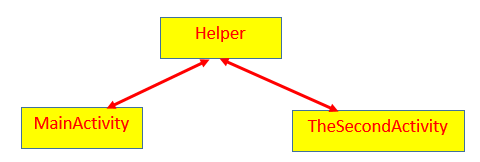Two activity classes and the helper class must be created in the same package. We can do this easily by clicking `app > Java` and choosing the package that contains the MainActivity.kt file in the right side window of Android Studio.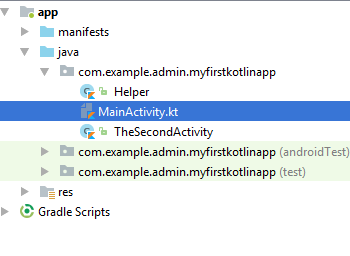Right-click on this package and choose either `New > Activity > Empty Activity`   if you want to create an activity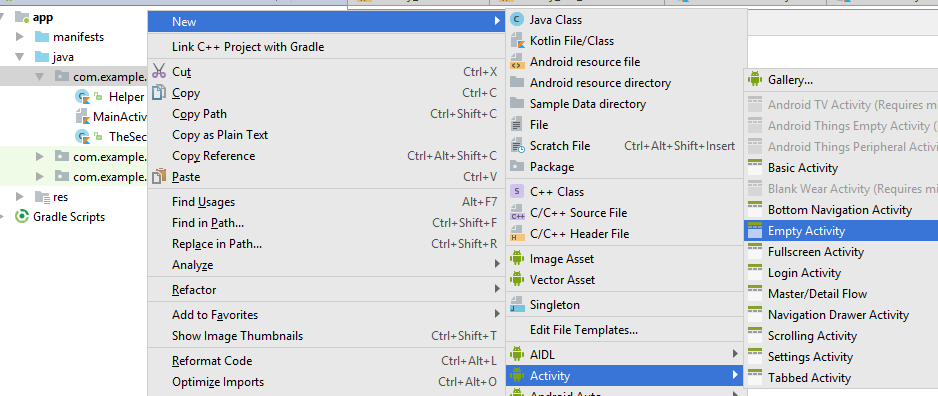or choose `New > Kotlin File/Class`   if you want to create a Kotlin class: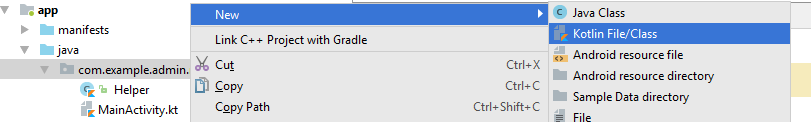In this application, two activity classes and the helper class are put in the same package named  `com.example.admin.myfirstkotlinapp`:Some controls are used in activities, as follows:

 Activity Control ID MainActivity Button display TheSecondActivity ButtonTextView backtxt

To move from an activity to another activity, we can use the  `Intent ` object and the  `startActivity ` method. The following code will demonstrate how to move from the MainActivity to the TheSecondActivity:

``val intent = Intent(this,TheSecondActivity::class.java) startActivity(intent)``

and we also move from the TheSecondActivity to the MainActivity:

``val intent = Intent(this,MainActivity::class.java) startActivity(intent)``

In the MainActivity.kt file, we define an interface and classes as follows:

``interface iShape { //properties var fillColor:String var outlineColor:String //methods fun Draw():String } class Shape (s:iShape):iShape by s class Circle:iShape{ override var fillColor:String = "" override var outlineColor: String = "" override fun Draw():String { return "I am a Circle. My Fill is \$fillColor and My Outline is \$outlineColor" } } class Rectangle:iShape{ override var fillColor:String = "" override var outlineColor: String = "" override fun Draw():String { return "I am a Rectangle. My Fill is \$fillColor and My Outline is \$outlineColor" } } class Square:iShape by Rectangle()``

Creating some shape objects and adding them into an  `ArrayList ` collection:

``val lst = ArrayList<Shape>() val rect = Shape(Rectangle()) rect.fillColor ="RED" rect.outlineColor ="BLUE" lst.add(rect) val circle = Shape(Circle()) circle.fillColor ="YELLOW" circle.outlineColor ="GRAY" lst.add(circle)``

``for(i:Int in 0..lst.size-1) Helper.DisplayString = (i+1).toString()+". "+ lst[i].Draw()``

In the TheSecondActivity activity, we will display information of shape objects through the helper class:

``txt.text = Helper.DisplayString``

In the Helper.kt file, we create the  `Helper ` class:

``````class Helper {
companion object {
var DisplayString: String = ""
set(value) {
field += value + "\n"
}
}
}``````

We have used `companion object` in the  `Helper ` class, and this way, we can access public members of the  `Helper ` class through the class name, as follows:

``txt.text = Helper.DisplayString``

Now, we can run the app, and our main activity has only a button:Click the DISPLAY button and we will move to the second activity, as follows: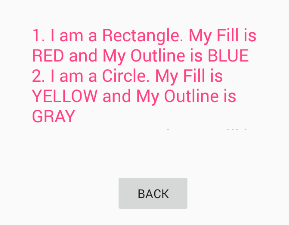We can click the BACK button to come back the main activity.

## Conclusion

So far, my series has had 5 parts. If you have not seen the previous parts, you can link to the series:

Part 1

Part 2

Part 3

Part 4

The source code of all the parts is shared here.

Topics:
kotlin, android, tutorial, oop, mobile, mobile app development

Opinions expressed by DZone contributors are their own.